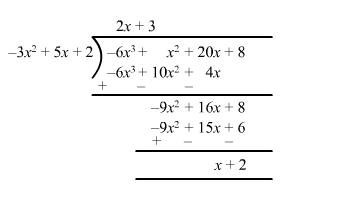Deepak Scored 45->99%ile with Bounce Back Crack Course. You can do it too!

# Verify division algorithm for the polynomials

Question:

Verify division algorithm for the polynomials $f(x)=8+20 x+x^{2}-6 x^{3}$ and $g(x)=2+5 x-3 x^{2}$

Solution:

We can write $f(x)$ as $-6 x^{3}+x^{2}+20 x+8$ and $g(x)$ as $-3 x^{2}+5 x+2$Quotient $=2 x+3$

Remainder $=x+2$

By using division rule, we have

Divided = Quotient × Divisor + Remainder

$\therefore-6 x^{3}+x^{2}+20 x+8=\left(-3 x^{2}+5 x+2\right)(2 x+3)+x+2$

$\Rightarrow-6 x^{3}+x^{2}+20 x+8=-6 x^{3}+10 x^{2}+4 x-9 x^{2}+15 x+6+x+2$

$\Rightarrow-6 x^{3}+x^{2}+20 x+8=-6 x^{3}+x^{2}+20 x+8$